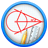GeometryQuantitative Aptitude Questions and Answers section on “Geometry” with solution and explanation for competitive examinations such as CAT, MBA, SSC, Bank PO, Bank Clerical and other examinations.

If △ABC is an isosceles triangles with ∠C = 90° and AC = 5 cm, then AB is:

If △ABC is an isosceles triangles with ∠C = = 90° and AC = 5 cm, then AB is: [A]$latex 5\sqrt{2}cm$ [B]$latex 2.5 cm$ [C]$latex 5 cm$ [D]$latex 10 cm$ Show Answer $latex \mathbf{5\sqrt{2}}cm$ AC = BC = 5 cm ∴ AB $latex =\sqrt{AC^{2}+BC^{2}}$ $latex = \sqrt{5^{2}+5^{2}} = \sqrt{50} = 5\sqrt{2} cm$ Hence option [A] ..